## Journal of Physics Research and Applications

All submissions of the EM system will be redirected to Online Manuscript Submission System. Authors are requested to submit articles directly to Online Manuscript Submission System of respective journal.

Editorial, J Phys Res Appl Vol: 2 Issue: 1

# On the Metric Structures and Connection Considerations of Gravitational Field in Finsler Spaces

Stavrinos PC1*, Ikeda S2 and Alexiou M3

1Department of Mathematics, University of Athens, Ilissia, Athens, Greece

2Ikeda Institute of Applied Geometry, Okayama-City, Japan

3Physics Division, National Technical University of Athens, Athens, Greece

*Corresponding Author : Panayiotis C Stavrinos
Department of Mathematics, University of Athens,Ilissia, Athens, Greece
Tel: (30)2107276416
E-mail: pstavrin@math.uoa.gr

Received: March 12, 2018 Accepted: March 14, 2018 Published: March 20, 2018

Citation: Stavrinos PC, Ikeda S, Alexiou M (2018) On the Metric Structures and Connection Considerations of Gravitational Field in Finsler Spaces. J Phys Res Appl 2:1.

## Abstract

In this paper, some physico-geometrical considerations are developed on the concept of nonlocal field theory associated with the Finslerian gravitational field. In particular, the nonlocalization by the internal variable at the more higher-order level or the more microscopic level than the Finslerian one is considered in detail. An application of the metric structure of Friedmann - Robertson - Walker - model (FRW) is also given in the framework of the vector bundle.

## Introduction

The last ten years the interest in the study of Applications of Finsler geometry to the general relativity, field theory and Cosmology has increased among the scientific community of Theoretical Physicists Different topics of elementary particles of gravitation and cosmology based on the Riemannian geometry are extended in the field Finsler geometry [1-33]. Especially metric models of locally anisotropic form e.g. as Finsler-Randers or Finsler-Randers-like metrics have played an important role in the evolution of structure of the gravitational field,for the Standard Model Extension (SME), Lorentz violation,cosmology [7,18]. Furthermore the concept of osculation between Riemann and Finsler space-time  was introduced by Stavrinos and contributed to provide a more connecting form in the study of subjects of general relativity and cosmology in this field, supported in the framework of Riemann-Finsler geometry [5,7].

In the framework of unified description of Finslerian gravitational field Ikeda developed the concept of nonlocalization which is embodied by the internal variable associated with each point of a manifold . Namely, the nonlocalized gravitational field is nonlocalized by attaching the internal variable to each point of the Einstein’s gravitational field. Therefore, the nonlocalized gravitational field has some “non”-Riemannian spatial structure owing to the internal variable. The Finslerian gravitational field is one typical example of the nonlocalized gravitational field, which is nonlocalized by the internal vector y = (yi, i = 1,2,3,4) annexed to each point x = (xλ ,λ = 1,2,3,4), that is to say the Finslerian gravitational field is the total space of internal and external field for each point-x and fibre-y on the vector bundle.

In a more general consideration the non-locality can be produced attatching to a point p of a base manifold M k a scalar or a spinor [35-37]. The concept of nonlocality is introduced to the field theory in order to supply a unified description of elementary particles.

In this paper, after describing the nonlocalized spatial structure of the Finslerian gravitational field, we shall consider some kinds of nonlocalizations of the gravitational field by adopting different internal variables. In particular, we shall consider, in detail, the nonlocalization at the more higher order level (or the more microscopic level) than the Finslerian one. We also introduce the form of nonlinear connection in a FRW-spacetime in the total space of the vector bundle.

Some applications of nonlinear connection are given for different types of Finsler spacetime. As well we present considerations of nonlocalization for a Kawaguchi space in connection with a spinorial structure.

## On the Finslerian Gravitational Field

As mentioned in Section 1, the Finslerian gravitational field is nonlocalized by the internal vector y associated with each point x. The vector y constitutes the so-called internal space or the fibre at each point x of the Einstein’s gravitational field, so that the Finslerian gravitational field is regarded as a unified field between the external (x) -field spanned by points {x} of the base manifold and the internal (y) -field spanned by vectors {y} in the internal space. In this consideration the metric tensor gij (x,y) is given in each fibre and comes from a differentiable fundamental function F: TM\{0} → R of one-degree homogeneous in the second variable y,

F(x, λy) =λF(x,y), λ>0, y=dx/dt [38,39]. The metric in the fibre has the form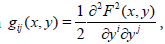which from physical considerations can be degenerate one. From a vector bundle-like view , the Finslerian gravitational field is identified with the total space of the vector bundle whose base manifold is the (x) -field and the fibre at the point x is the (y)- field.

In the total space, the following adapted frame is set properly:(1)

where A=(λ,i)=1,2,3,…,8 anddenotes the nonlinear connection. A nonlinear connection (N-connection) lives on the total space of the tangent bundle of a manifold M and it can be introduced in the same manner as a linear connection [38,39]. It induces a parallel transport on the base manifold. Locally, a N-connection is defined by a Whitney sum on the tangent space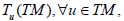where HuTM denotes the horizontal subbundle and VuTM the vertical one. Locally the coefficients of N-connection are given bywithThe adapted frame is made to be adapted to the direct-sum structure of the metrical structure GAB of the total space, where(2)gλu and gij represent the metrics on the base manifold and of fibres. The connection relation is represented by the metrical connection coefficients, wherewith(3)

Namely, the covariant derivatives are defined, for example, for an arbitrary vector V(x,y) and have the form(4)

The metrical conditions are considered by gλμ|v = 0, gλμ| = 0 and gij|l=0 and. As is understood from the above, the y-dependence of the Finslerian gravitational field is caused by the nonlocalization with respect to y. In the following, we shall consider some other kinds of nonlocalizations by adopting different kinds of internal variables and generalized or specialized structures of the Finslerian structure mentioned above.

## On the Nonlocalization-I

First, if we take another vector z = (zα: α=1,2,3,4) as the internal variable, besides y, then we can generalize the Finslerian structure by using two nonlinear connectionsas follows:(5)metrical structure:(6)

connection relation: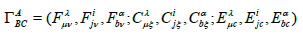(7)

covariant derivatives:(8)In the above formulas, if yp= (yi, y0) (y0is an independent scalar) that belongs to a 5-dimensional space, we can adopt it, instead of yi or zα, then (5) - (8) are reduced to those formulas for this case, where in (5) - (8), zα, zbare replaced by y0 and the indices α, b,… are replaced by 0. This is adjusted to the time-dependent Lagrange geometry with space-time (3+1) dimensions [40-43]. In this case the form of consideration is reduced to the tangent bundle-like one with y0 being the time (y0 ≡ t) . On the other hand, if we choose only y0 as the independent internal variable, then the relations (1) - (4) are reduced to the following formulas:(9)

A generalized Finsler - Randers model can be introduced from the metric (2)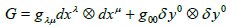(10)If we apply this study for a cosmological model Friedman- Robertson-Walker (FRW) then the metric of FRW can be extended to the form of the total space.(11)

In the above relation we have replaced the first term of the equation (10) with the metric of FRW-model and we have put g00=1. In the case of y0 = t0 = const the rel. (11) is reduced in a spacelike formThe Christoffel symbols of FRW model are given by(12)In this case the connection coefficients in the total space can be written aswherewithChristoffel symbols of FRW metric. In the above mentioned case the covariant derivative of the vector V(x,y) is given by(14)

## Applications of Non-linear Connection

Some physical meanings of y0 and Na0 have already been considered in  and for the C000 in cosmology .

1. In the classical Kaluza-Klein theory regarding x0 as the 5th coordinate and set g00 = 1 in the rel.(10), then we can find that the nonlinear connection N0λ plays the role of electromagnetic potential. In this case, the torsion tensor defined byrepresents the electromagnetic field tensor .

2. The Finsler-Randers (F-R) metric is given by F(x,y)=(aij(x)yiyj)1/2 + uiyiwith aij(x) pseudo-Riemannian metric and um represents a weak primordial vector field, where ÇuiÇ « 1 The time component u0 of um is incorporated in the geometry of space-time by Cartan’s torsion tensor C000 with the relation. If we substitute this relation to (14) we getfrom the last relation we notice that the covariant derivative of V0 depends on u0. A physical interprentation of the non-linear connection for the model of Finsler-Randers space with a type of rotation of angular momenta of galaxies can be given by(15)

where γijk are the Christoffel symbols for the Riemannian spacetime and Akm means antisymmetrization and substruction between the indices k and m. A form of nonlinear connection for the FRWcosmological model in a FR space can be presented by substituting the values of the relation (12) in the relation (15). The Cartan nonlinear connection for a FRW model has the formwhere γijk take the values from (12). By using Cartan’s nonlinear connection in the relation (15) we get(16)

The form of the adapted frame of the rel.(5) becomes(17)

whereare the local coefficients of Cartan nonlinear connection and zk an internal variable as the yi .

3. A weak nonlinear connection can be given in a F-R model by using a weak Riemannian metric of the form aij(x)=nij + hij(x), Ç hij Ç=1, where nij, represents the Minkowski metric and hij(x) a weak perturbation. The corresponding weak nonlinear connectionfor a F-R space-time is written because of (15) as(18)

withthe weak Christoffel symbols.

4. In the F-R cosmological model the rotation of a vector u from an axis can âβ be given in virtue of a rotation group. In this case, the nonlinear connection can be written in the form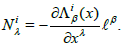It expresses the variation of the rotation group with respect to the anisotropic axis âβ . The rotation group can be used giving a profound geometrical and physical meaning to the concept of nonlinear connection N. The Berwald type form of the nonlinear connection Ni λ is given byThe tensor fielddenotes the curvature of nonlinear connection and expresses a torsion of variation of the rotation group Λik . Non-linear connections have also been considered in the framework of a spinor theory for Finsler spaces [45,46].

## On the Nonlocalization-II

Now, we shall consider the nonlocalization at the higher order level than the Finslerian one. First, if another vector Z(= zα = 1,2,3,4) is taken into account at the one more microscopic level, then the form of consideration is generalized to the second order vector bundlelike form. In this case, the relations (5) - (8) are generalized to the following formulas:(19)

metrical structure: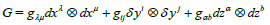(20)

connection relation:(21)(22)

Along this line, we can adopt two or more vectors at the second order or third order or more higher order level, if necessary. The formulas are generalized in the same manner as in (19) - (22) by replacing (x,y,z) with (x,y,z1,z2), (x,y,z1,z2,z3),…, where many kinds of nonlinear connections and connection coefficients must be introduced. Similar with the previous consideration in 3 for the metric and the connection of FRW its form can be extended because of nonlocalization (20) - (22) in the framework of a higher order form.

## On the Nonlocalization-III

Kawaguchi space and spinor connections

From a more physical viewpoint, if we adopt the more microscopic internal variables other than the vectors such as the spinor,etc. which do not obey the coordinate transformation, then we must take into account the order space-structure higher than the Finslerian space-structure. An analogous but different case has been studied in [28,36,46].

The higher order space (or Kawaguchi space) of order M(=1,2,3,…) is a metrical space in which an arc length along a curve x=x(t) is given by the integral(23)

Where L means the fundamental function andplays the role of internal variable in our sense. Of course, this space is regarded as a generalized Riemann or Finsler space nonlocalized by x(n), (x(n)(n ≥ 2) is not a vector). The Riemann space is the higher order space of order 0 and the Finsler space is the higher order space of order 1. Therefore, the nonlocalization in this section must be considered within the framework of the higher order space of order M ≥ 2, where the independent variable must be replaced by. (x,y,z≡x(n)) (n ≥ 2; y≡x(1))

In this case, δy and δz represent the base connections defined by (for a certain n ≥ 2)(24)

whereare introduced to determine the isomorphisms between the elements ofand those ofandbeing the spaces attached to the generalized line-elementsandrespectively. The relation (24) can be formally reduced to the same forms as in (19) under some convenient conditions such as,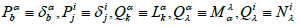but the contents of those quantities are different from each other intrinsically. Those base connections should be determined from a physical viewpoint, by the intrinsic behaviour of y and z .

As to the metrical structure and the connection relation for the case of (x,y,z=x(n)) they should be formulated within the framework of the higher order space of order M ≥ 2, which, however, cannot be written in similar and simple forms as in (20) - (22). But, from a physical viewpoint if z = x(n) ,(n >2) is likened to the spinor ψ and (x, ψ) is chosen as the independent variable then (19) - (22) can be reformulated as follows(25)

where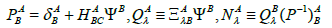In this case the metric is given by(26)

with connections and covariant derivatives(27)(28)

Further, if we take up a nonlocalized spinor field Ï (x, ψ) then we can define its covariant derivatives in the form(29)

whereandandA, B represent the spinor indices. Those quantities such as Θλ and GA mean spin affine connections playing the role of spin gauge fields obeying the principle of minimal coupling.

As is understood from the above, our method to nonlocalization can be applied to any internal variable, even if there exist many different kinds of internal variables. One can also extend the content of previous mentioned theories and apply them for connections and metrics on Lorentzian manifolds and Lorentzian metrics on the tangent bundle of a Finsler space-time based on non-linear connections. Furthermore applications of null spacetime can be generalized in the geometry of the total space of a null congruence manifold which were considered in other works for a Lorentzian manifold .

This theme will be examined in an upcoming paper.

## References